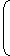## Permutations and Combinations

1. Factorial Notation:
Let n be a positive integer. Then, factorial n, denoted n! is defined as:
n! = n(n - 1)(n - 2) ... 3.2.1.
Examples:
1. We define 0! = 1.
2. 4! = (4 x 3 x 2 x 1) = 24.
3. 5! = (5 x 4 x 3 x 2 x 1) = 120.
2. Permutations:
The different arrangements of a given number of things by taking some or all at a time, are called permutations.
Examples:
1. All permutations (or arrangements) made with the letters abc by taking two at a time are (abbaaccabccb).
2. All permutations made with the letters abc taking all at a time are:
( abcacbbacbcacabcba)
3. Number of Permutations:
Number of all permutations of n things, taken r at a time, is given by:
 nPr = n(n - 1)(n - 2) ... (n - r + 1) = n! (n - r)!
Examples:
1. 6P2 = (6 x 5) = 30.
2. 7P3 = (7 x 6 x 5) = 210.
3. Cor. number of all permutations of n things, taken all at a time = n!.
4. An Important Result:
If there are n subjects of which p1 are alike of one kind; p2 are alike of another kind;p3 are alike of third kind and so on and pr are alike of rth kind,
such that (p1 + p2 + ... pr) = n.
 Then, number of permutations of these n objects is = n! (p1!).(p2)!.....(pr!)
5. Combinations:
Each of the different groups or selections which can be formed by taking some or all of a number of objects is called a combination.
Examples:
1. Suppose we want to select two out of three boys A, B, C. Then, possible selections are AB, BC and CA.
Note: AB and BA represent the same selection.
2. All the combinations formed by abc taking abbcca.
3. The only combination that can be formed of three letters abc taken all at a time is abc.
4. Various groups of 2 out of four persons A, B, C, D are:
AB, AC, AD, BC, BD, CD.
5. Note that ab ba are two different permutations but they represent the same combination.
6. Number of Combinations:
The number of all combinations of n things, taken r at a time is:
 nCr = n! = n(n - 1)(n - 2) ... to r factors . (r!)(n - r)! r!
Note:
1. nCn = 1 and nC0 = 1.
2. nCr = nC(n - r)
Examples:
 i.   11C4 = (11 x 10 x 9 x 8) = 330. (4 x 3 x 2 x 1)
 ii.   16C13 = 16C(16 - 13) = 16C3 = 16 x 15 x 14 = 16 x 15 x 14 = 560. 3! 3 x 2 x 1

### Example:

In how many ways a committee, consisting of 5 men and 6 women can be formed from 8 men and 10 women?

Required number of ways= (8C5 x 10C6)= (8C3 x 10C4)
 =8 x 7 x 6 x 10 x 9 x 8 x 73 x 2 x 1 4 x 3 x 2 x 1
= 11760.

### Example:

In how many ways can the letters of the word 'LEADER' be arranged?

The word 'LEADER' contains 6 letters, namely 1L, 2E, 1A, 1D and 1R.Required number of ways = 6! = 360. (1!)(2!)(1!)(1!)(1!)

### Example:

From a group of 7 men and 6 women, five persons are to be selected to form a committee so that at least 3 men are there on the committee. In how many ways can it be done?

We may have (3 men and 2 women) or (4 men and 1 woman) or (5 men only).Required number of ways= (7C3 x 6C2) + (7C4 x 6C1) + (7C5)
 =7 x 6 x 5 x 6 x 5+ (7C3 x 6C1) + (7C2) 3 x 2 x 1 2 x 1
 = 525 +7 x 6 x 5 x 6+7 x 63 x 2 x 1 2 x 1
= (525 + 210 + 21)
= 756.

### Example:

How many 3-digit numbers can be formed from the digits 2, 3, 5, 6, 7 and 9, which are divisible by 5 and none of the digits is repeated?

Since each desired number is divisible by 5, so we must have 5 at the unit place. So, there is 1 way of doing it.
The tens place can now be filled by any of the remaining 5 digits (2, 3, 6, 7, 9). So, there are 5 ways of filling the tens place.
The hundreds place can now be filled by any of the remaining 4 digits. So, there are 4 ways of filling it.Required number of numbers = (1 x 5 x 4) = 20.

### Example:

In how many different ways can the letters of the word 'MATHEMATICS' be arranged so that the vowels always come together?

In the word 'MATHEMATICS', we treat the vowels AEAI as one letter.
Thus, we have MTHMTCS (AEAI).
Now, we have to arrange 8 letters, out of which M occurs twice, T occurs twice and the rest are different.Number of ways of arranging these letters = 8! = 10080. (2!)(2!)
Now, AEAI has 4 letters in which A occurs 2 times and the rest are different.
 Number of ways of arranging these letters = 4! = 12. 2!Required number of words = (10080 x 12) = 120960.

#### Unknown

Do You Like This??? Then Hit Subscribe Button. You Will Get Every Post, Which Is Worth Reading